Select Page

# Matrices 12 Science Maths CBSE Answers for MCQ in English

Matrices 12 Science Maths CBSE Answers for MCQ in English to enable students to get Answers in a narrative video format for the specific question.

Expert Teacher provides Matrices 12 Science Maths CBSE Answers for MCQ through Video Answers in English language. This video solution will be useful for students to understand how to write an answer in exam in order to score more marks. This teacher uses a narrative style for a question from Matrices not only to explain the proper method of answering question, but deriving right answer too.

Please find the question below and view the Answer in a narrative video format.

Question:

## Similar Questions from CBSE, 12th Science, Maths, Matrices

Question 1 : Let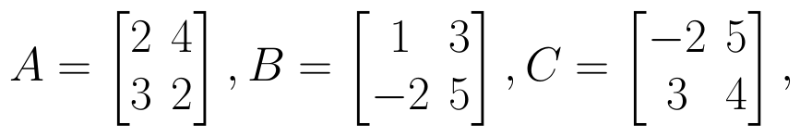Find 3A - C. (View Answer Video)

Question 2 : Compute: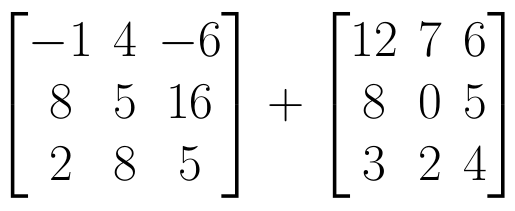. (View Answer Video)

Question 3 : Find the value of x, if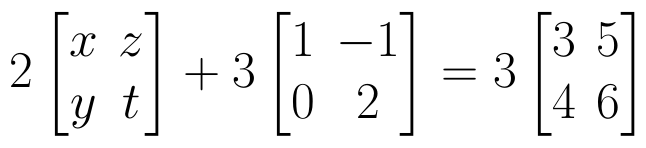. (View Answer Video)

Question 4 : Find the value of X, if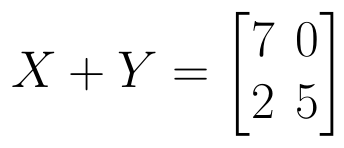and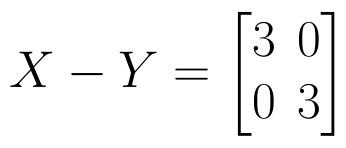. (View Answer Video)

Question 5 : Given,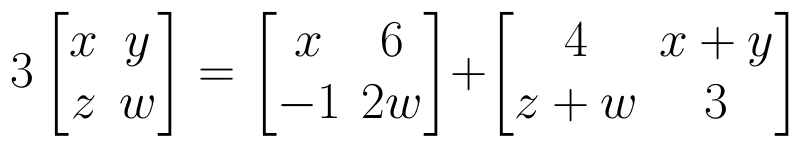, find the value of x. (View Answer Video)

### Determinant

Question 1 : If A =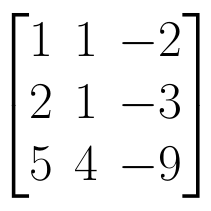, find | A |. (View Answer Video)

Question 2 :  Using the properties of determinants, evaluate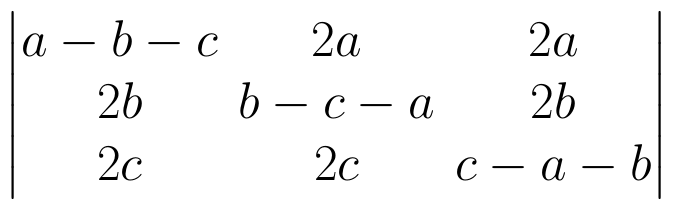. (View Answer Video)

Question 3 : Evaluate  the determinants in :.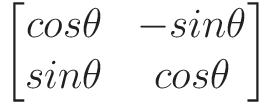(View Answer Video)

Question 4 : If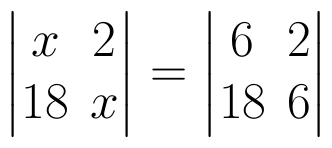, then x is equal to, (View Answer Video)

Question 5 : Evaluate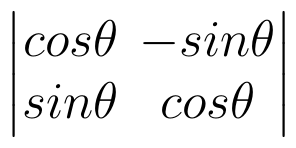. (View Answer Video)

### Application of Integrals

Question 1 : Find the area of the region bounded by the curve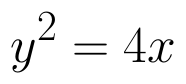and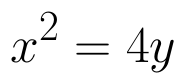. (View Answer Video)

Question 2 : Find the area bounded by curves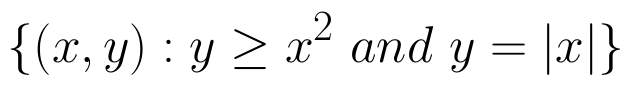. (View Answer Video)

Question 3 : Find the area of the smaller part of the circle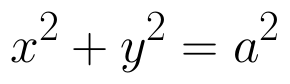cut off by the line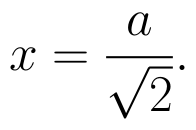(View Answer Video)

Question 4 :

Find the area of the given curves and given lines: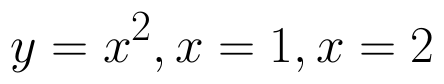and x-axis

Question 5 : Find the area bounded by the curve y = sin x between x = 0 and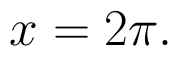(View Answer Video)

### Vector Algebra

Question 1 :  P and Q are two points with position vectors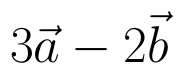and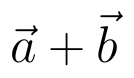respectively. Write the position vector of a point R which divides the line segment PQ externally in the ratio 2:1.  (View Answer Video)

Question 2 :  If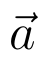and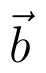denote the position vectors of points A and B respectively and C is a point on AB such that AC = 2 CB, then write the position vector of C.  (View Answer Video)

Question 3 : Find a unit vector parallel to the sum of vectors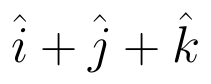and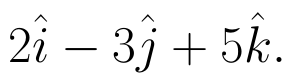(View Answer Video)

Question 4 : Find a vector in the direction of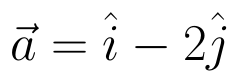that has magnitude 7 units.   (View Answer Video)

Question 5 : Compute the magnitude of the following vectors: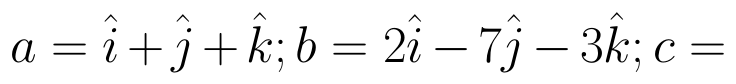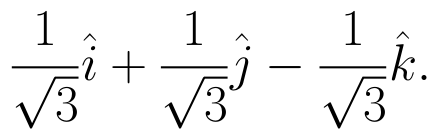(View Answer Video)